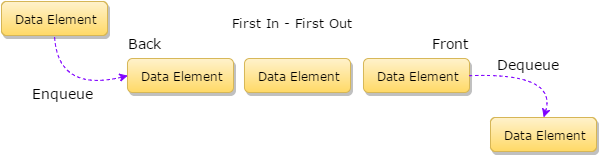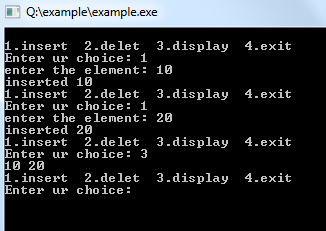# Concepts of Queue in Data Structure

The queue is a linear data structure used to represent a linear list. It allows insertion of an element to be done at one end and deletion of an element to be performed at the other end.

In this chapter, you will be given an introduction to the basic concepts of queues along with the various types of queues which will be discussed simulating the real world situation.

## What is a Queue?

A queue is a linear list of elements in which deletion of an element can take place only at one end called the front and insertion can take place on the other end which is termed as the rear. The term front and rear are frequently used while describing queues in a linked list. In this chapter, you will deal with the queue as arrays.

In the concept of a queue, the first element to be inserted in the queue will be the first element to be deleted or removed from the list. So Queue is said to follow the FIFO (First In First Out) structure. A real-life scenario in the form of example for queue will be the queue of people waiting to accomplish a particular task where the first person in the queue is the first person to be served first.Other examples can also be noted within a computer system where the queue of tasks arranged in the list to perform for the line printer, for accessing the disk storage, or even in the time-sharing system for the use of CPU. So basically queue is used within a single program where there are multiple programs kept in the queue or one task may create other tasks which must have to be executed in turn by keeping them in the queue.

## Queue as an ADT (Abstract Data Type)

The meaning of an abstract data type clearly says that for a data structure to be abstract, it should have the below-mentioned characteristics:

• First, there should be a particular way in which components are related to each other
• Second, a statement for the operation that can be performed on elements of abstract data type must have to be specified

Thus for defining a Queue as an abstract data type, these are the following criteria:

• Initialize a queue to be empty
• Check whether a queue is empty or not
• Check whether a queue is full or not
• Insert a new element after the last element in a queue, if the queue is not full
• Retrieve the first element of the queue, if it is not empty
• Delete the first element in a queue, if it is not empty

## Representation of Queue as an Array

Queue is a linear data structure can be represented by using arrays. Here is a program showing the implementation of a queue using an array.
Example:

``````#include <iostream>
#include<stdlib.h>
using namespace std;

class queuearr {
int queue1;
int rear, front;

public:
queuearr()
{
rear = -1;
front = -1;
}
void insert(int x)
{
if (rear > 4) {
cout << "queue over flow";
front = rear = -1;
return;
}
queue1[++rear] = x;
cout << "inserted " << x;
}

void delet()
{
if (front == rear) {
cout << "queue under flow";
return;
}
cout << "deleted " << queue1[++front];
}

void display()
{
if (rear == front) {
cout << " queue empty";
return;
}
for (int i = front + 1; i <= rear; i++)
cout << queue1[i] << " ";
}
};

int main()
{
int ch;
queuearr qu;
while (1) {
cout << "\n1.insert 2.delet 3.display 4.exit\nEnter ur choice: "; cin >> ch;
switch (ch) {
case 1:
cout << "enter the element: "; cin >> ch;
qu.insert(ch);
break;
case 2:
qu.delet();
break;
case 3:
qu.display();
break;
case 4:
exit(0);
}
}
}
``````

Output: